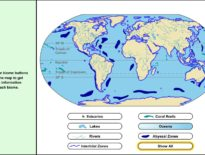# High School Math Learning Activity: Graphing Imaginary Numbers

Imaginary numbers can be difficult to comprehend. Visualizing the problem often makes it easier to understand.

Use this great tool to practice graphing imaginary numbers.

This interactive activity is embedded in our High School Math course.

Students develop algebraic fluency by learning the skills needed to solve equations and perform manipulations with numbers, variables, equations, and inequalities. They also learn concepts central to the abstraction and generalization that algebra makes possible. Students learn to use number properties to simplify expressions or justify statements; describe sets with set notation and find the union and intersection of sets; simplify and evaluate expressions involving variables, fractions, exponents, and radicals; work with integers, rational numbers, and irrational numbers; and graph and solve equations, inequalities, and systems of equations. They learn to determine whether a relation is a function and how to describe its domain and range; use factoring, formulas, and other techniques to solve quadratic and other polynomial equations; formulate and evaluate valid mathematical arguments using various types of reasoning; and translate word problems into mathematical equations and then use the equations to solve the original problems.

Request a free catalog of K-12 Courses

Request the Scope & Sequence for a course

## High School Math Learning Activity: Graphing Imagi…